##1. Conjugacy of two points/lines

Given a circle (c), two points {X, Y} are said to be conjugate with respect to (c) when the polar of one passes through the other. This is a symmetric relation and if we restrict it on an arbitrary line (e) it defines an involutive homography on the line Y = F(X) (see HomographicRelation.html ).
Analogously is defined the conjugacy of two lines: Two lines {x,y} are said to be conjugate with respect to the circle (c), when each passes from the pole of the other.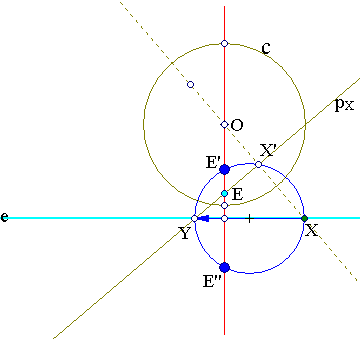The symmetry of the relation is due to the reciprocity of poles-polars: if Y is on the polar of X, then also X is on the polar of Y. If X' is the projection of X on its polar pX, triangle XX'Y has a right angle at X' and {X,X'} are inverse (see Inversion.html ) with respect to circle (c).
From this follows that all circles with diameter XY are orthogonal to (c) and being also orthogonal to line (e) belong to the bundle of circles (II) which is orthogonal to the circle bundle (I) generated by {c, e}.
The kind of the circle-bundle (II) depends on the position of line (e) relative to the circle c.
 If line (e) does not intersect the circle c, then bundle (II) is of intersecting type. All the circles of (II) pass through two fixed points {E',E''} on the line orthogonal to (e) through the center O of the circle. This line is the common radical axis of all circles of the bundle (II). Points {E', E''} are harmonic conjugate with respect to {O, E}, E being the pole of (e).
In this case the involution F has no fixed points on line (e).
 If line (e) intersects the circle (c), then bundle (II) is of non-intersecting type with limit points the intersection points {X0, X1} of the circle (c) with line (e).
In this case the involution F has two fixed points on line (e) which are precisely {X0, X1}.

##2. Conjugacy with respect to a conic

Since the idea of polar generalizes naturally to a conic one can repeat the definition in this more general context and define conjugacy of points with respect to a conic: Two points {X,Y} are said to be conjugate with respect to a conic, if the polar of one passes through the other. Again the relation is symmetric and defines an involutive homography Y=F(X) on the points of line e.
The definition of line-conjugacy can be transferred also verbatim to this general case.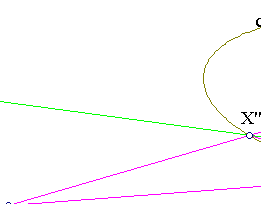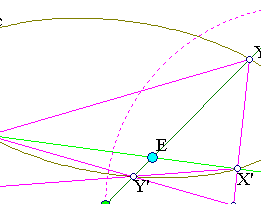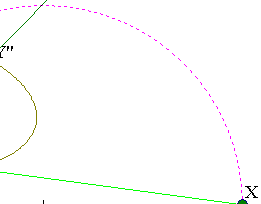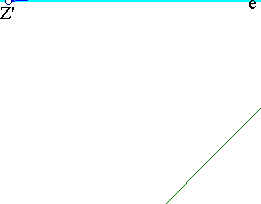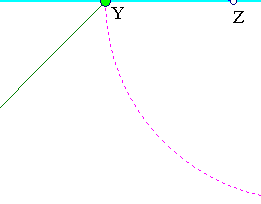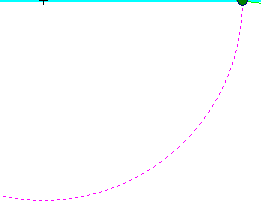The above basic figure shows two points {X,Y} on line (e) conjugate with respect to a conic c. Point E is the pole of (e) and lines {Y'Y'', X'X''} are respectively the polars of {X, Y}. By Pascal's theorem the opposite sides of quadrangle X'Y'X''Y'' intersect at two points {Z, Z'} which are on (e) and are also related by the homographic relation Z'=F(Z). Besides {Z,Z'} are harmonic conjugate with respect to {X,Y}.
The previous statements on the circle bundle (II) of circles with diameters XY transfer almost unchanged to this case. The general figure can be transformed by an appropriate projectivity to a circle (c') and a line (e'), the homography relation being preserved, we obtain also a map of the bundle (II) here to the corresponding bundle of {c', e'}. We conclude that the circle bundle in the present general case is of intersecting type or non-intersecting type in dependence of the position of the line e to the conic c:
 If the line (e) does not intersect the conic then the bundle (II) of circles with diameters XY is of intersecting type. All circles pass through two fixed points {E',E''}, which can be explicitly constructed from the data.
The involutive homgoraphy Y = F(X) has no fixed points in this case.
 If the line (e) intesects the conic (c) at two points {X0, X1} then the circle bundle (II) is of non-intersecting type and has these two points as limit points. The same points are also fixed points of the involutive transformation F.

##3. The intersecting type

Here are some remarks concerning case  of the previous section. In this case line (e) does not intersect the conic and bundle (II) of circles with diameters XY is of intersecting type. All circles pass through two points {E',E''} which are symmetric with respect to (e) and can be determined from the pair {c,e}.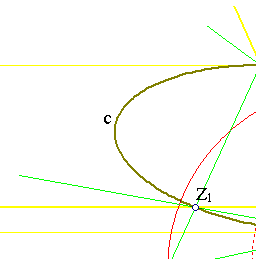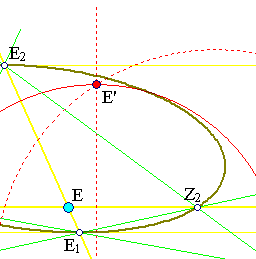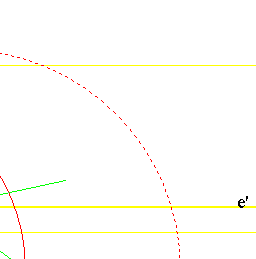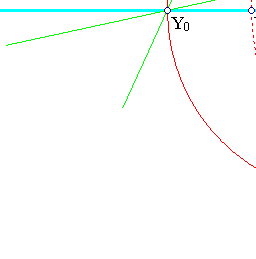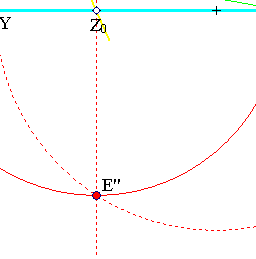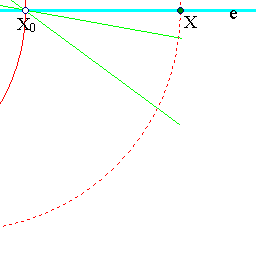Because {Z,Z'} are harmonic conjugate to {X,Y} the center of the circle cX with diameter XY never coincides with Z except in the case in which ZY = ZX, in which, by the harmonic conjugacy Z' falls to infinity, hence Z'E, which is the polar of Z, becomes parallel to e.
 The previous position of the center of the circle is thus the pole Z0 of the line e' through E and parallel to (e).
 Another interesting circle of the circle-bundle (II) results when X obtains the position of Z0. This corresponds to an interchange of the roles of (X,Y) and (Z,Z') and represents the case in which Y is at infinity, {Z,Z'} are symmetric with respect to X and circle cX is a line through Z0.
 The previous arguments show that Z0 is the trace on (e) of the common radical axis of all circles of the bundle, hence the line carying {E',E''}. The points can then be easily determined from the constancy of the product Z0X*Z0Y.
 Points {E', E''} can though be constructed also geometrically by noticing the relation of pairs of points {Z,Z'} and {X,Y} in the figure of section 2. Namely one pair is defined by the diagonals of a quadrangle inscribed in the conic whereas the other is defined by the common points of pairs of opposite sides. Applying this remark to the pair consisting of Z0 and the point at infinity of line e we find the other points {X0,Y0} symmetric with respect to Z0 through the easily constructible figure shown.
 From what has been said {E1,E2} are the contact points of tangents to c which are parallel to line (e). Thus line E1E2 passes through the center of the conic (if one exists).

##4. Conjugate diameters in central conics

Last remark of previous section is crucial in considering central conics and allowing the line (e) to move parallel to itself. Points {E1, E2} remain fixed while if line (e) moves to infinity its pole E tends to the center of the conic. In that case also points {Z1, Z2} tend to the extremities of the diameter which is conjugate to the diameter E1E2 of the conic and also parallel to line e. In the limit, when e identified with the line at infinity the involution F becomes the usual conjugation of diameters of the conic.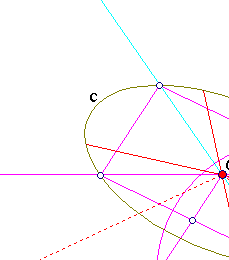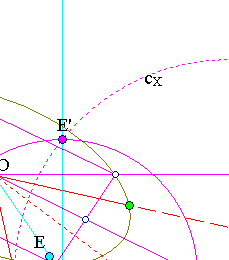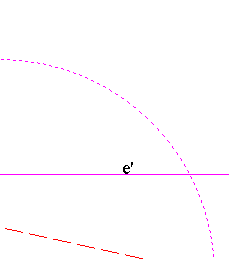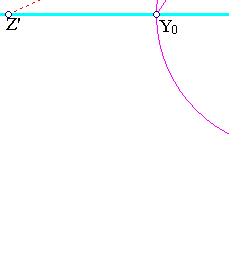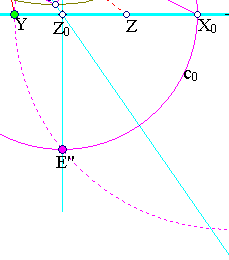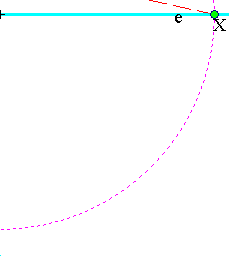To study this involution (of conjugacy of diameters in a central conic) we identify the line at infinity with the pencil O* of lines through O. Then our involution becomes an involution of this pencil and can be studied by taking the traces X, Y , ... of lines through O on a fixed line e. This new involution associates to each point X of line (e) a point Y such that {OX, OY} are conjugate diameters of the conic. There is again a naturally associated pair of conjugate points {Z,Z'} to {X,Y} comming from the diameters parallel to the sides of the parallelogram with diagonals along {OX, OY}. By analogous arguments as in the previous section we can identify the common points of the circle bundle of circles cX having diameter XY.
 Z0 is, as before, the intersection point of line OE with line (e). E being again the pole of (e).
 Points {X0,Y0} identifying the minimal circle of bundle (II) are the intersection with two diameters along lines {OX0, OY0} which are parallel to the sides of the parallelogram inscribed in the conic and having diagonals along lines OZ0 and the parallel (e') to (e) through O.

##5. Orthogonal diameters

The previous section delivers a recipe by which we can determine the two orthogonal to each other diameters of a central conic (along lines {OX1, OY1} in the figure below).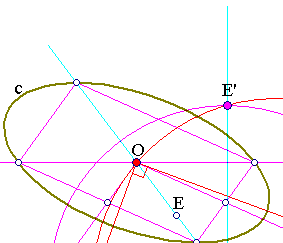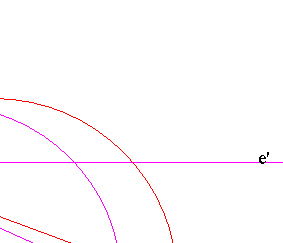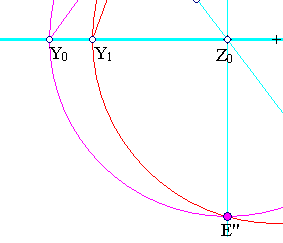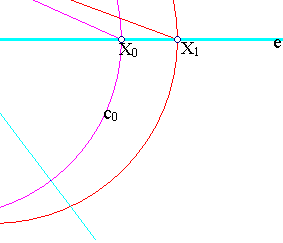Take a line (e) non intersecting the conic and consider its pole E.
 Find point Z0 by intersecting (e) with line OE (O center of the conic).
 Find points {X0,Y0} by constructing the parallelogram with diagonals along lines OZ0, and the parallel (e') to (e) through O. Then projecting O parallel to the parallelogram-sides on line (e).
 Determine points {E', E''} of the circle c0 with diameter X0Y0.
 Draw the circle c1 which passes through points {O, E', E''}. This circle determines through its intersections {X1, Y1} with line (e) the two orthogonal conjugate directions of the conic.